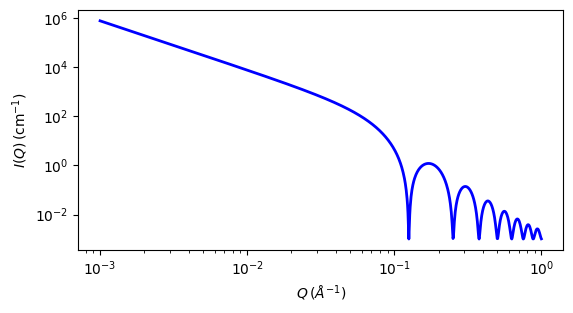# lamellar¶

Lyotropic lamellar phase with uniform SLD and random distribution

Parameter

Description

Units

Default value

scale

Scale factor or Volume fraction

None

1

background

Source background

cm-1

0.001

thickness

total layer thickness

50

sld

Layer scattering length density

10-6-2

1

sld_solvent

Solvent scattering length density

10-6-2

6

The returned value is scaled to units of cm-1 sr-1, absolute scale.

Polydispersity in the bilayer thickness can be applied from the GUI.

Definition

The scattering intensity $$I(q)$$ for dilute, randomly oriented, “infinitely large” sheets or lamellae is

$I(q) = \text{scale}\frac{2\pi P(q)}{q^2\delta} + \text{background}$

The form factor is

$P(q) = \frac{2\Delta\rho^2}{q^2}(1-\cos(q\delta)) = \frac{4\Delta\rho^2}{q^2}\sin^2\left(\frac{q\delta}{2}\right)$

where $$\delta$$ is the total layer thickness and $$\Delta\rho$$ is the scattering length density difference.

This is the limiting form for a spherical shell of infinitely large radius. Note that the division by $$\delta$$ means that $$scale$$ in sasview is the volume fraction of sheet, $$\phi = S\delta$$ where $$S$$ is the area of sheet per unit volume. $$S$$ is half the Porod surface area per unit volume of a thicker layer (as that would include both faces of the sheet).

The 2D scattering intensity is calculated in the same way as 1D, where the $$q$$ vector is defined as

$q = \sqrt{q_x^2 + q_y^2}$Fig. 46 1D plot corresponding to the default parameters of the model.¶

Source

lamellar.py

References

1. F Nallet, R Laversanne, and D Roux, J. Phys. II France, 3, (1993) 487-502

2. J Berghausen, J Zipfel, P Lindner, W Richtering, J. Phys. Chem. B, 105, (2001) 11081-11088

Authorship and Verification

• Author: